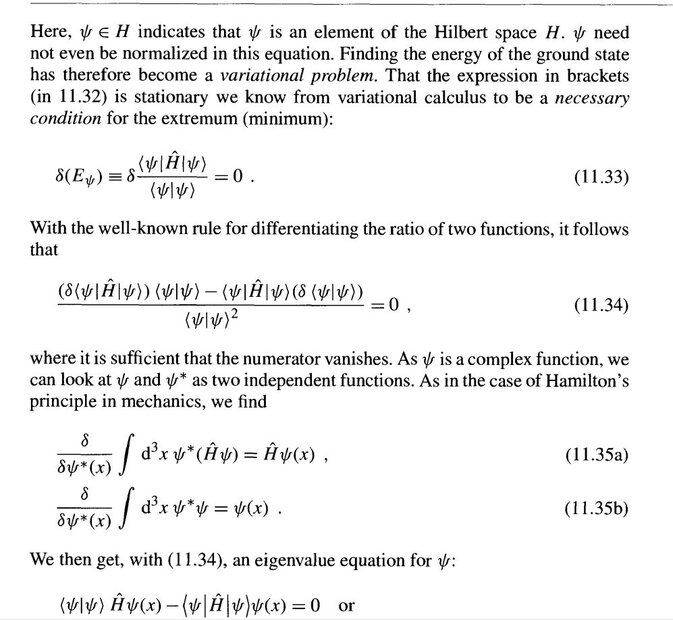# Schrodinger Equation from Ritz Variational Method

• I
• Samama Fahim

#### Samama Fahim(This is from W. Greiner Quantum Mechanics, p. 293 from the topic of Ritz Variational Method)

1) Are ##\frac{\delta}{\delta \psi^{*}}## derivatives in equations 11.35a and 11.35b? If this is so, we can differentiate under the integral sign to get ##\int d^3x (\hat{H}\psi)## in equation 11.35a and ##\int d^3x \psi## in 11.35b, but why would ##\int d^3x (\hat{H}\psi)## be equal to just ##\hat{H}\psi## and ##\int d^3x \psi## to just ##\psi##?

2) Moreover, we replace ##\delta \bra{\psi}\hat{H}\ket{\psi}## in 11.34 with ##\hat{H}\psi## and ##\delta \bra{\psi}\ket{\psi}## with ##\psi## resulting in the equation at the bottom. Why is that? Is it that ##\delta (\psi^{*}\hat{H}\psi) = \hat{H}\psi \delta \psi^{*}## because we are looking at variation in ##\psi^{*}## and so we can take ##\hat{H} \psi## out?

•